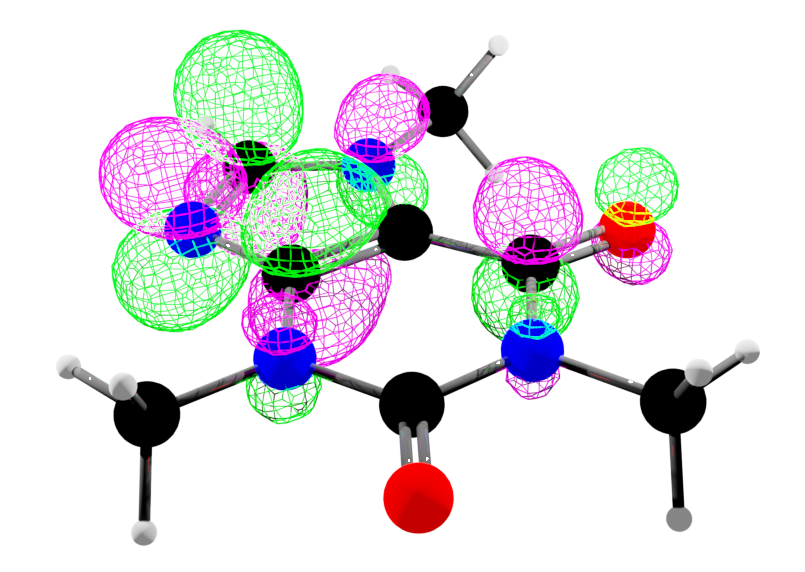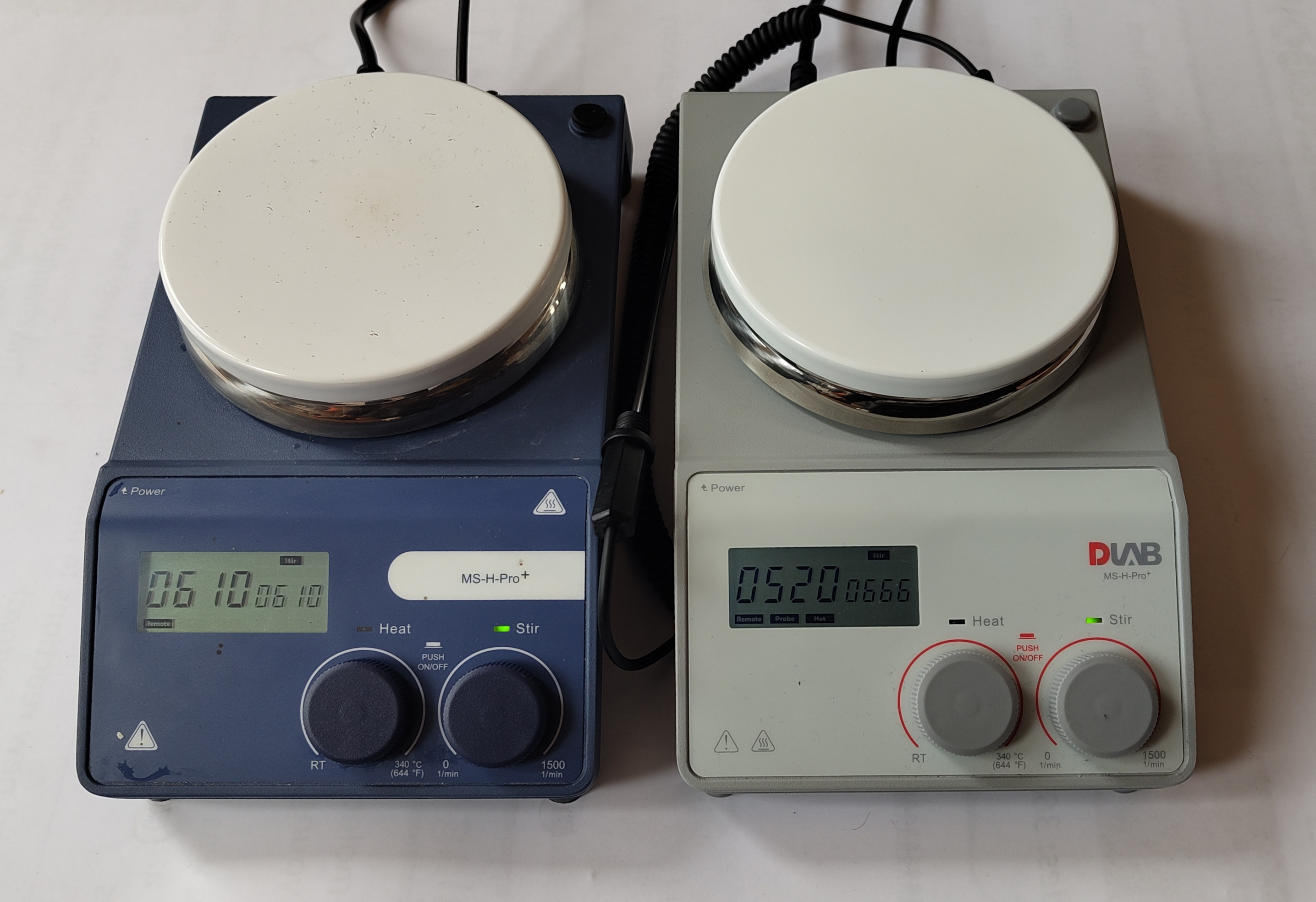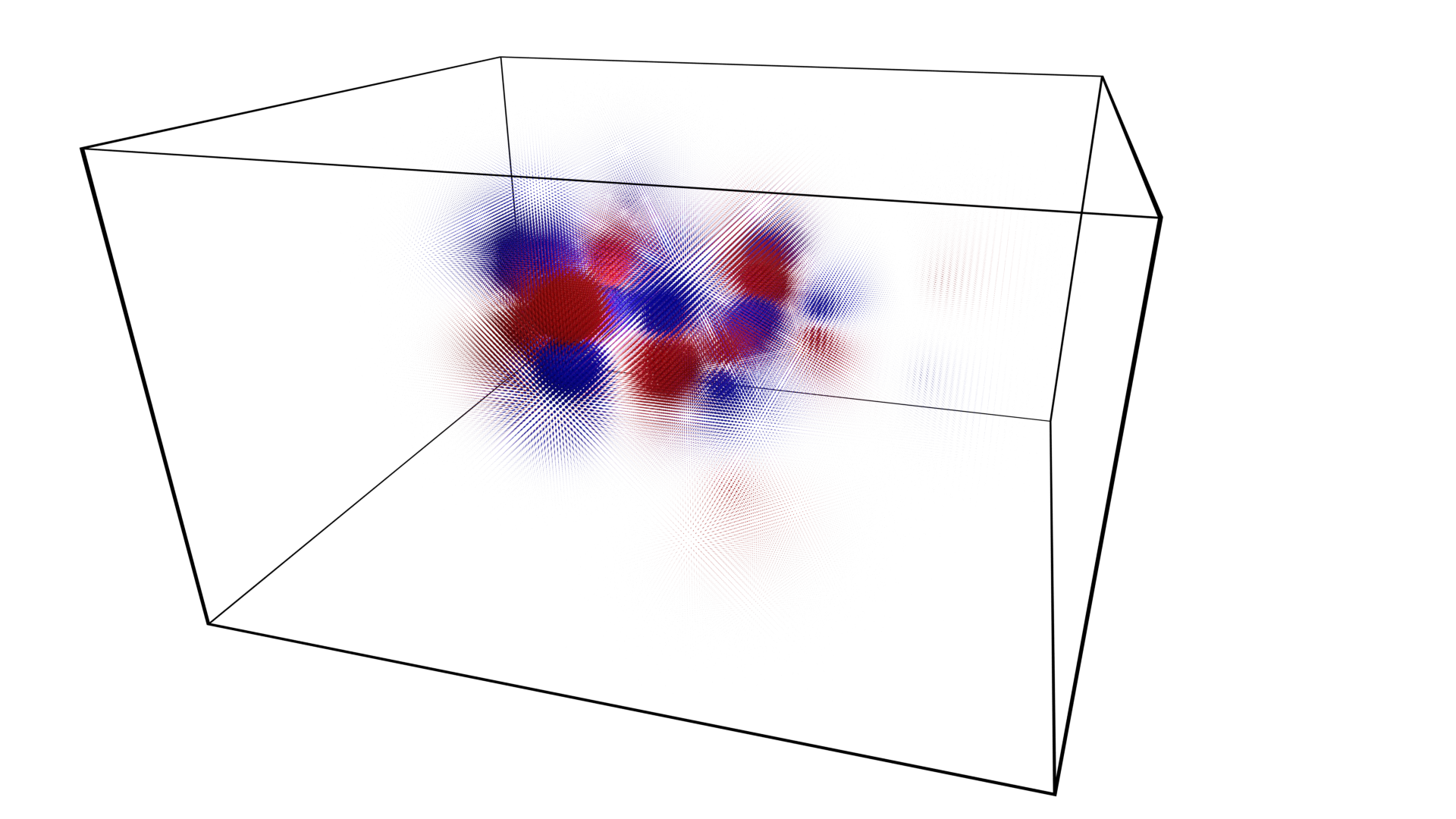# Marching NumPy

GitHub

The visualisation of numeric data is a key component of computer graphics.
Data is often encountered as numeric values distibuted over a regular space - sometimes volume data are called voxels data. Visualisation of this numeric data is key to the interpretation and/or appreciation of the data. For example:

• medicine: medical imaging such as MRI and CT scanners.
• science: quantum mechanical calculations and data recording.
• cartography: linking data such as height, land usage, etc to map coordinates.
• entertainment: generation of artwork generated terrain and visualsation of metaballs.

An important method for the visualisation of volume data is to calculate an isosurface: a mesh surface that follows as closely as possible where the data crosses a certain level threshold.

This package includes an implementation of the Lorensen Marching Cubes isosurface calculation algorithm using only Python and NumPy methods: `MarchingCubesLorensen.marching_cubes_lorensen()`. The algorthimn follows these processes:

• Calculate Intersects. For each time that the volume value crosses the level threshold along each axis, interpolate the position of the intersection - this becomes a vertex for the output mesh. Also calculate a unique id for that vertex.
• Assign Types. Consider each corner of the volume unit and whether it is above or below the level threshold. Assign a unique identifier to each unit based upon the outcome of these logical binary tests. For a cube there are 8 vertices that gives 256 possible outcomes [that are related to a set of 15 core geometries by symmetry].
• Look Up Geometry. Use the calculated type to index a precalculated geometry table the defines how the calculated vertices should be joined using triangular geometry.

Example usage:

```>>> import numpy
>>> import MarchingNumPy
>>> vertices,triangles = MarchingNumPy.marching_cubes_lorensen(volume, level=0.05)
>>> vertices.shape
(4138, 3)
>>> triangles.shape
(8244, 3)
```

The image below - a molecular orbital of caffeine (pink and green wireframe) - was generated in Blender using the output of this function.This volume data `test_volume.npy` is from the Cubefile package.

# Hotplates

GitHub

The MSHPro is low-cost hotplate stirrer.The hotplate has a RS232 9-pin connector on the rear that allows control of its functions. This package is a tool for control of these hotplates via serial interface.

This package is an interface to all commands provided by the `Hotplates.MSHPro` class.
Commands are avilable to get information and control the hotplate's speed and temperature.
All the formal communication structure is described by the `Hotplates.MSHProCommunication` module.

Example usage:

```>>> import Hotplates
>>> hp = Hotplates.MSHPro(port="/dev/ttyUSB0")
>>> hp.status()
{'success': True, 'stir_set': 'Off', 'stir_actual': 0, 'heat_set': 'Off', 'heat_actual': 17.5, 'stir_on': False, 'heat_on': False, 'heat_limit': 340.0}
>>> hp.stir(400)  # Wait after command for hotplate to reach speed
>>> hp.status()
{'success': True, 'stir_set': 400, 'stir_actual': 399, 'heat_set': 'Off', 'heat_actual': 17.7, 'stir_on': True, 'heat_on': False, 'heat_limit': 340.0}
>>> hp.off()
>>> hp.status()
{'success': True, 'stir_set': 'Off', 'stir_actual': 0, 'heat_set': 'Off', 'heat_actual': 1.1, 'stir_on': False, 'heat_on': False, 'heat_limit': 340.0}
```

Serial communication is full duplex and this is acheived using `Hotplates.SerialThreadedDuplex.Serial`, an extension of PySerial's `serial.Serial`.

# Cubefile

GitHub

.cube files are generated from quantum mechanical chemistry calculations. They contain data about the atoms of a molecule: their element, charge and position, as well as volume data from molecular orbital calculations - where you can expect to find electrons within a molecule.

This package is a tool for loading data into Python from a .cube file.
See MarchingNumPy for a method of converting this volume data to an isosurface.

Example usage:

```>>> import Cubefile
>>> cf = Cubefile.Cubefile("_testfiles/caffeine_54.cube")
>>> cf.voxels.shape
(111, 98, 64)
```

The package includes some example .cube data calculated for caffeine that has been rendered as a point cloud below.